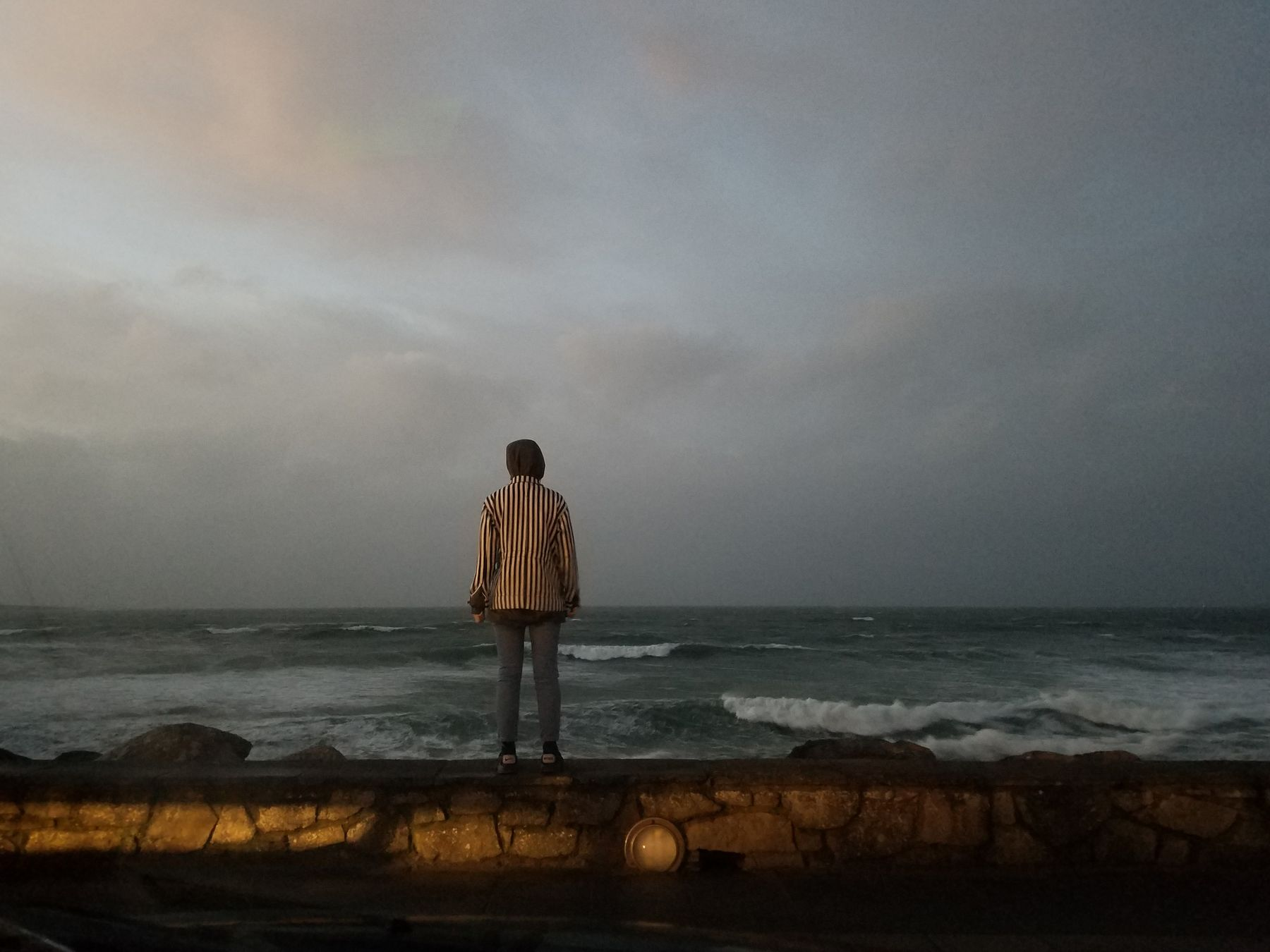0:00/???

From the recording Vol 1

Illustration by Mariana Rockwell. Instagram @memar_

## Lyrics

This is the House

This is the house where I was transformed
Where once was a serpent
Where once was a slave

And this is the room where the martyrs were burned
Where once was a gallows
Where once was a grave

This is the house where I was desired
Where once was a stranger
Where once was a stray

And this is the room where the nightmares were sired
Where once was a warning
Where once was a way

Put bars on the windows
Put locks on the door
And tell the dear children
Don’t come round no more.

This is the house where I was absolved
Where once was a heathen
Where once was a whore

And this is the room where the shadows were called
Where once was a circle
Where once was a star

This is the house where I was devoured
Where once was a hunter
Where once was a horn

And this is the room where the bodies were stored
Where once was an orgy
Where once was a war